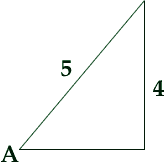Subject: Double Angle Identities
Date: Fri, 18 Jun 1999 20:08:54 -0600

Name: Ryan Grade 12 student

If sinA = 4/5, and A is a first quadrant angle, find sin4A

Hi Ryan
 From the right triangle in the diagram with sin A = 4/5, Pythagoras' Theorem shows that the third side of the triangle has length 3 and hence cos A = 3/5. Use the exprression sin 2A = 2 sin A cos A to calculate sin 2A. Now start again. Since you know sin 2A use Pythagoras' Theorem to find cos 2A and hence calculate sin 4A. Cheers HarleyGo to Math Central

To return to the previous page use your browser's back button.